# 数据分析三大类型

• 探索型数据分析
• 验证型数据分析
• 预测型数据分析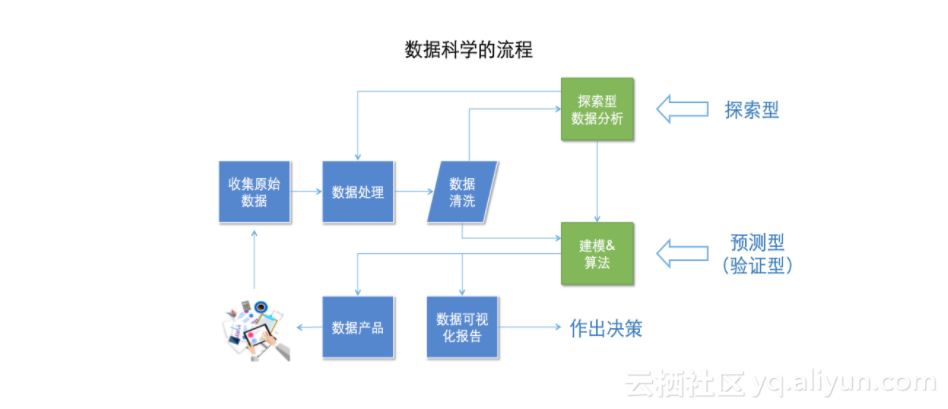## 探索型数据分析的作用

• 与数据清理相辅相成
• 支持验证型数据分析、预测型数据分析

## 探索型数据分析的常用图表

• 条形图、直方图
• 饼图（饼图在探索型数据分析中使用较少，原因是肉眼对于角度之间的大小差别没有对高度之间的差别敏感）
• 折线图、散点图
• 箱形图

## 下面仔看一些例子

import seaborn
%matplotlib inline
tips = seaborn.load_dataset("tips")
#bar 条形图
seaborn.barplot(x="day",y="total_bill",data=tips)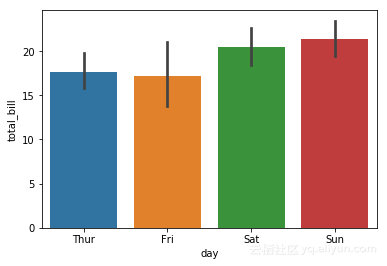#dist 直方图
#这里只有一个参数X，是要分析的数据，比如学生的成绩
seaborn.distplot(X)
#line chart 折线图
seaborn.pointplot(x="day",y="tip",data=tips,ci=68)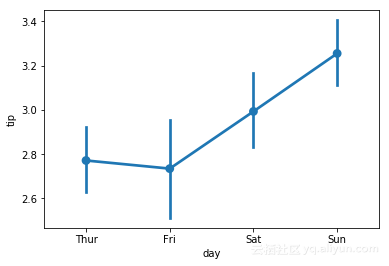#scatter diagram 散点图
seaborn.regplot(x="total_bill",y="tip",data=tips)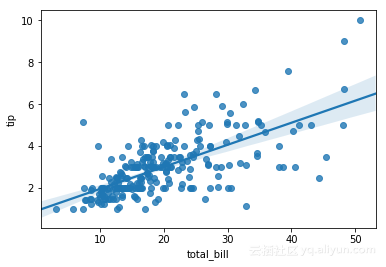#box plot 箱形图
seaborn.boxplot(x="day",y="total_bill",data=tips)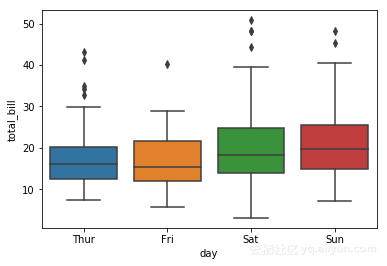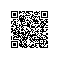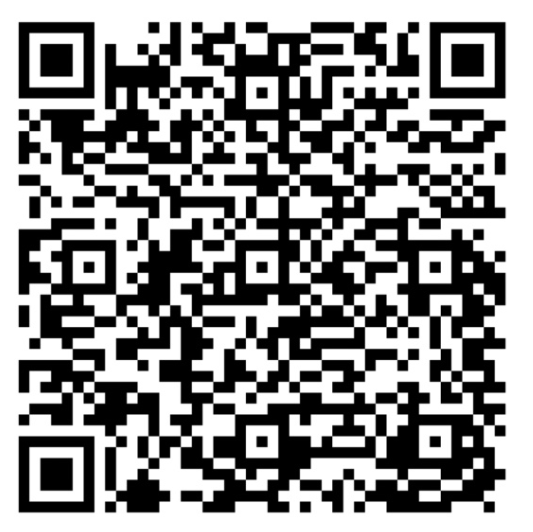使用钉钉扫一扫加入圈子
+ 订阅